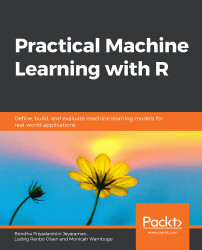•#### Practical Machine Learning with R#### Overview of this book

With huge amounts of data being generated every moment, businesses need applications that apply complex mathematical calculations to data repeatedly and at speed. With machine learning techniques and R, you can easily develop these kinds of applications in an efficient way. Practical Machine Learning with R begins by helping you grasp the basics of machine learning methods, while also highlighting how and why they work. You will understand how to get these algorithms to work in practice, rather than focusing on mathematical derivations. As you progress from one chapter to another, you will gain hands-on experience of building a machine learning solution in R. Next, using R packages such as rpart, random forest, and multiple imputation by chained equations (MICE), you will learn to implement algorithms including neural net classifier, decision trees, and linear and non-linear regression. As you progress through the book, you’ll delve into various machine learning techniques for both supervised and unsupervised learning approaches. In addition to this, you’ll gain insights into partitioning the datasets and mechanisms to evaluate the results from each model and be able to compare them. By the end of this book, you will have gained expertise in solving your business problems, starting by forming a good problem statement, selecting the most appropriate model to solve your problem, and then ensuring that you do not overtrain it.Free Chapter
An Introduction to Machine LearningData Cleaning and Pre-processingFeature EngineeringIntroduction to neuralnet and Evaluation MethodsLinear and Logistic Regression ModelsUnsupervised LearningAppendix## Introduction

We learned about the process of machine learning in Chapter 1, An Introduction to Machine Learning, and looked at the different ways to process data in Chapter 2, Data Cleaning and Pre-processing. In this chapter, we will delve deep into the feature engineering process. Feature engineering is a process in which we select the attributes that are related to the target field in our dataset. The selection is made using techniques such as correlation analysis, Principal Component Analysis (PCA), and other techniques. During this process, new features can also be generated that are meaningful and add information to our dataset. In addition to this, we can generate statistics of existing numeric fields as features, as they contain statistical information about the fields or attributes.

In this chapter, we will learn how to create features for date variables, time series data, strings, and numeric variables, and explore text features. Furthermore, we will look at the implementation...